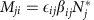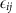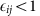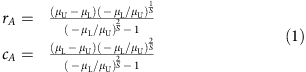Thank you for visiting nature.com. You are using a browser version with limited support for CSS. To obtain the best experience, we recommend you use a more up to date browser (or turn off compatibility mode in Internet Explorer). In the meantime, to ensure continued support, we are displaying the site without styles and JavaScript.

Predicting the stability of large structured food webs

Abstract

The stability of ecological systems has been a long-standing focus of ecology. Recently, tools from random matrix theory have identified the main drivers of stability in ecological communities whose network structure is random. However, empirical food webs differ greatly from random graphs. For example, their degree distribution is broader, they contain few trophic cycles, and they are almost interval. Here we derive an approximation for the stability of food webs whose structure is generated by the cascade model, in which ‘larger’ species consume ‘smaller’ ones. We predict the stability of these food webs with great accuracy, and our approximation also works well for food webs whose structure is determined empirically or by the niche model. We find that intervality and broad degree distributions tend to stabilize food webs, and that average interaction strength has little influence on stability, compared with the effect of variance and correlation.

Introduction

The stability of large ecological systems has been investigated for more than 40 years1. The interest in this subject was sparked by a short article by Robert May1, who was able to show that large ecosystems with random interactions would invariably be unstable, with potential consequences for biodiversity maintenance. To obtain this result, May employed basic tools of random matrix theory, and recent advances in this area2,3 allowed for an extension of May's result to more general cases4,5—effectively identifying the main drivers of stability in ecological communities.

All these findings hinge on an important assumption that the network structure describing who interacts with whom in an ecosystem is random4,5, that is, any two species have the same probability of interacting, irrespective of species identity. However, the empirical food webs collected thus far display major departures from the structure of random graphs6. For example, in empirical webs the degree distribution, describing the number of partners each species interact with, is much broader6 than in random graphs; the webs contain only a handful of trophic cycles7 (in which, for example, species a consumes b, b consumes c and c consumes a), while random graphs with the same number of links would contain many more; finally, empirical webs are almost interval—there is a way to order all species such that consumers tend to prey on consecutive species in the hierarchy8.

To overcome this limitation, we derive an approximation for the stability of food webs whose structure is generated by the cascade model9, which assumes that species can be ordered such that ‘larger’ species consume ‘smaller’ ones. We sample the strength of interaction between consumers and resources from an empirical distribution, obtained via body-size scaling theory5. We show numerically that our approximation estimates the stability of these food webs with great accuracy, and that similar results are obtained when we generate food webs starting from empirical data, or when using the niche model10. We show that intervality and broad degree distributions tend to stabilize food webs, and we highlight a counterintuitive result: although research on the relationship between stability and the distribution of interaction strengths has historically focused on average strength11,12,13,14, we show that its role in determining stability is small, compared with that of variance and correlation.

Results

Constructing the community matrix

We want to determine the real part of the leading (‘rightmost’) eigenvalue of the community matrix M, Re(λM,1), which is the key for the local asymptotic stability of the ecological system. In fact, the community matrix1,15 determines the effects of one species on another around a feasible equilibrium point: if all the eigenvalues of M have a negative real part, the equilibrium is locally stable—small perturbations will be buffered (Supplementary Note 1). The study of community matrices has a long history in ecology, but so far methods relying on large random matrices4,5 have not been able to account for realistic food web structure, and were based on the simplifying assumption of a completely random network, in which every species has the same probability of consuming any other.

Here we study the matrix M constructed in the following way. First, an S × S adjacency matrix K is built according to the cascade model9: the species are ordered from 1 to S, and each species j has probability C of consuming each of the preceding species. A coefficient Kij=1 means that species i is consumed by j. Then, we build the community matrix M by independently sampling each pair of coefficients (Mij,Mji)i<j from the bivariate distribution Z=(X,Y) whenever Kij=1. For simplicity, we leave Mii=0. Setting all diagonal coefficients to −d would simply shift all the eigenvalues (λi=λid), and sampling the diagonal coefficients from a distribution with mean −d and a given variance would yield qualitatively the same results, provided that the variance is not large compared with that of the off-diagonal coefficients5. In this setting, we can think of Re(λM,1) as the minimum amount of self-regulation we would have to impose on each species to make the system stable11.

Because the pairs (Mij,Mji) model the effect of the consumer on the resource (Mij) and that of the resource on the consumer (Mji), we have Mij<0 and Mji>0 whenever Kij=1. Thus, we assume Z=(X,Y) to be a bivariate distribution with marginal means μx and μy, Var(X)=σx2, Var(Y)=σy2, and Cov(X, Y)=ρxyσxσy, where μx<0, and μy>0 (see Methods section).

The matrix M then contains non-positive coefficients in the upper-triangular part (either 0 when Kij=0, or negative when Kij=1). Similarly, the lower-triangular part of the matrix contains only non-negative coefficients. We denote by μU and μL the means of the upper- and lower-triangular coefficients; by σU2 and σL2 the variances; and by ρULσUσL the covariance.

Derivation strategy

Having shown how M is built, we now illustrate the strategy we use to find the distribution of its eigenvalues. First, we decompose the matrix into the sum of two matrices, M=A+B, where A is a deterministic matrix whose upper-triangular coefficients are all equal to μU, and all the lower-triangular to μL. B is obtained by difference, B=MA, and therefore its coefficients are described by a bivariate distribution with means 0 and covariance matrix identical to that found for the coefficients of M. Matrix A models the ‘signal’, and B the ‘noise’ (Fig. 1). We then studied the spectrum of A and B separately.

For A, one can show (see Methods section) that all the eigenvalues fall on the curve describing a circle in the complex plane with center (cA,0) and radius rA. When −μU>μL, that is, negative effects are stronger than positive ones, the bulk of the eigenvalues of A have positive real part, and a few eigenvalues with large modulus have negative real part (Fig. 1). In this case, Re(λA,1)≈rA+cA, and 0≤Re(λA,1)≤−μU for any choice of size and parameters (Supplementary Note 3).

For σL2=σU2=σ2, the eigenvalues of B would follow the elliptic law3, and thus, for large S, they would be approximately uniformly distributed over an ellipse in the complex plane centred at (0,0), with horizontal semi-axis approximately (2)1/2(1+ρ) and vertical semi-axis approximately (2)1/2(1−ρ). Here we conjecture that even in the more general case of σL2σU2, the eigenvalues of B are approximately uniform in an ellipse centred at (0,0) with horizontal semi-axis rh,B, and vertical semi-axis rv,B (see Methods section).

Having shown that when −μU>μL, Re(λ A,1)≈rA+cA, and Re(λB,1)≈rh,B, we take the last approximation: Re(λM,1)≈Re(λA,1)+Re(λB,1). In fact, the eigenvalues of M fall either close to the curve found for A, or in the ellipse found for B, centred at (Re(λA,1),0) instead of (0,0) (Fig. 1). This type of approximation is known to be accurate for symmetric matrices (following Weyl’s inequality16), but our results suggest that it is well suited for the matrices studied here as well, provided that the variances σL2 and σU2 are sufficiently large compared with μL and μU (Supplementary Note 3).

Numerical results

To test the quality of our approximation, we built 150 adjacency matrices K using the cascade model9. The size of the matrix was randomly chosen among {500, 750, 1,000}, and the probability of interaction C was sampled uniformly between 0.1 and 0.3. We sampled the pairs (Mij,Mji) independently from the empirical distribution Z whenever Kij=1. The results are presented in Fig. 2. The approximation is very accurate, and clearly superior to what expected following the derivations by May1 or Tang et al.5.

We then built 150 adjacency matrices using the niche model10, which can generate trophic cycles, so that there is no way to order the species such that all the coefficients in the upper-triangular part of M are non-positive. Hence, even knowing the distribution Z, we need to find a way to calculate μU, μL and so on. Clearly, the eigenvalues of M do not change when we sort the species in different ways, but, because our approximation makes explicit use of the coefficients in the upper- and lower-triangular parts of M, each ordering of the species would yield a different approximation. To choose the ‘best’ approximation, we sorted the species in the adjacency matrix K so that the minimum number of non-zero coefficients were present in the lower-triangular part of the matrix, and then used this order to parameterize the approximation (Supplementary Note 2). Results show that the approximation is also excellent for the niche model, even though the matrices built in this way are slightly more likely to be stable than those built using the cascade model (Fig. 2).

Finally, we took 15 large empirical food webs (Supplementary Note 2) and parameterized each food web 10 times. Also in this case, we sorted the adjacency matrix K to obtain the ‘most upper-triangular’ configuration prior to calculating the approximation. Despite the fact that empirical networks are quite different from those generated by the cascade model (for example, containing ‘modules’17 and having broader degree distributions6), the approximation is clearly superior to previous approaches, even though it still tends to over-estimate the actual Re(λM,1).

Effect of network properties

Having an analytic expectation for Re(λM,1) allows us to investigate which particular features of network structure are stabilizing. For example, the networks produced by the niche model differ from those generated by the cascade model in three main aspects. First, although trophic cycles are forbidden in the cascade model, the niche model typically produces networks with a handful of trophic cycles. Second, in a food web produced by the niche model, we can always order the species such that each predator consumes prey that are adjacent in the hierarchy (for example, this would be the case if each predator were to prey upon all the species falling in a certain interval of sizes18), a property known as ‘intervality’. Intervality is rarely attained by the cascade model, especially for large webs9. Finally, the degree distributions (that is, number of predators per prey, and number of prey per predator) differ substantially between the networks produced by the two models (starting from the same parameters).

To test whether these features can account for the small discrepancy between our expectation and that found in simulations, we built three variants of the cascade model: (i) a version of the cascade model producing the same degree distribution for the consumers as that of the niche model; (ii) a version producing interval food webs; (iii) a version yielding the same consumer degree distribution as the niche model, and producing interval food webs (that is, a cycle-less niche model). In Fig. 3 we show that all these modifications are slightly stabilizing, making the matrices built using these variants as likely to be stable as those for the niche model. Similarly, modifying the cascade model so that it matches the degree distribution of a given empirical network recapitulates the small deviation we observe between the predicted and observed Re(λM,1) for matrices generated using empirical food web structures (Supplementary Note 4).

The case of strong positive effects

So far, we have concentrated on the case of −μU>μL meaning that negative interactions are on an average stronger than the positive ones. This is what is typically found in food webs—due to the low efficiency of transformation of prey into predators. When this is not the case, the eigenvalue distribution of M is ‘flipped’ around the imaginary axis (that is, the distribution is like that in the bottom-left panel of Fig. 1, but with the x-axis reversed), such that a pair of large complex roots determines the stability of the system (Supplementary Note 3). This observation is sufficient to make a suggestive prediction: large systems in which the positive effects dominate the negative ones will likely lose stability through a Hopf bifurcation (two complex roots crossing the imaginary axis), typically giving rise to limit cycles. For a simple Lotka–Volterra model, a pair of coefficients of matrix M modelling a resource–consumer interaction can be written as Mij=−βijNi* and, where βij is the attack rate of j on i, Ni* is the equilibrium biomass of resource i, andis the conversion efficiency of resources into consumers. In this simple setting, given that, the Hopf bifurcations should be most common in the presence of an inverted biomass pyramid, typically occurring in planktonic19 or other aquatic20 systems. This prediction is quite suggestive, because in general it is not possible to predict the type of bifurcation simply looking at basic quantities such as μU and μL. Our hypothesis could be investigated both theoretically and empirically.

Discussion

The new approximation allows us to quantify the contribution of several key quantities to the stability of large food webs. Take a food web built by the cascade model for a given size S, connectance C and parameterized using the bivariate distribution Z=(X,Y) defined by its means μx and μy, its variances σx2 and σy2 and the correlation ρxy. In Fig. 4, we show how Re(λM,1) responds to changes in the parameters, by re-calculating the approximation when a given parameter is multiplied by a factor θ.

Interestingly, even doubling (or halving) the average interaction strengths, μx and μy has very little effect on the stability of the system. This is due to the fact that, when −μU>μL and Re(λA,1) is very constrained, and increasing the average strength of interaction simply makes the large eigenvalues with negative real part even more negative, with negligible effects on stability (note, however, that average strengths would be the most important quantities when −μU<μL; Supplementary Note 3). The size and connectance have a stronger effect (confirming the inverse relationship between stability and ‘complexity’21), but far less than the variances and the correlation, with increasing variances being strongly destabilizing, and high negative correlation being strongly stabilizing. These observations question a large body of literature11,12,13,14 focusing on the relationship between mean interaction strength and stability.

Here we have derived for the first time an analytic approximation able to predict the stability of large, structured food webs. The approach is based on the decomposition of the community matrix into the sum of two matrices, and the same approach could be used to study the influence of stability of other important food web properties, such as modularity17, the presence of trophic groups22 and the division into trophic levels.

Methods

Distribution of interaction strengths

We build an empirical distribution for Z using a large database detailing the relationship between consumer and resource body sizes for thousands of observed trophic interactions23. To transform body-size relationships into the coefficients of the community matrix, we need to estimate the interactions between species as well as the equilibrium biomasses for all populations. To this end, we make use of body-size scaling theory5 to construct a reasonable distribution Z (Supplementary Note 2). All the figures presented here are obtained for a particular choice of parameters, but our results hold also for alternative parameterizations, and even for entirely different distributions (Supplementary Note 4). In particular, the results are consistent with the universality property found for other random matrices2,3: once fixed the mean and covariance matrix, the details of the distribution of the coefficients have no effect on the distribution of the eigenvalues.

Spectrum of A and B

In Supplementary Note 3, we derive the eigenvalues of A. All eigenvalues fall on the curve describing a circle in the complex plane withwhere rA is the radius of the circle, and is (cA,0) its center.

For matrix B, we conjecture that its eigenvalues are approximately uniformly distributed in the ellipse in the complex plane with horizontal semi-axis rh,B≈(α+ρULσUσL(S−1))/(α)1/2 and vertical semi-axis rv,B≈(αρULσUσL(S−1))/(α)1/2, where α/S tends to (σU2σL2)/log(σU2/σL2) for S large (Supplementary Note 3). In the limit of σUσL, we obtain αS, consistently with the elliptic law.

How to cite this article: Allesina, S. et al. Predicting the stability of large, structured food webs. Nat. Commun. 6:7842 doi: 10.1038/ncomms8842 (2015).

References

1. 1

May, R. M. Will a large complex system be stable? Nature 238, 413–414 (1972).

2. 2

Tao, T., Vu, V. & Krishnapur, M. Random matrices: Universality of ESDs and the circular law. Ann. Probab. 38, 2023–2065 (2010).

3. 3

Nguyen, H. & O'Rourke, S. The elliptic law. Int. Math. Res. Notice doi: 10.193/imrn/rnu174 (2014).

4. 4

Allesina, S. & Tang, S. Stability criteria for complex ecosystems. Nature 483, 205–208 (2012).

5. 5

Tang, S., Pawar, S. & Allesina, S. Correlation between interaction strengths drives stability in large ecological networks. Ecol. Lett. 17, 1094–1100 (2014).

6. 6

Dunne, J. A., Williams, R. J. & Martinez, N. D. Food-web structure and network theory: the role of connectance and size. Proc. Natl Acad. Sci. USA 99, 12917–12922 (2002).

7. 7

Allesina, S., Alonso, D. & Pascual, M. A general model for food web structure. Science 320, 658–661 (2008).

8. 8

Stouffer, D. B., Camacho, J. & Amaral, L. A. N. A robust measure of food web intervality. Proc. Natl Acad. Sci. USA 103, 19015–19020 (2006).

9. 9

Cohen, J. E., Briand, F. & Newman, C. M. Community Food Webs: Data and Theory Springer (1990).

10. 10

Williams, R. J. & Martinez, N. D. Simple rules yield complex food webs. Nature 404, 180–183 (2000).

11. 11

de Ruiter, P. C., Neutel, A.-M. & Moore, J. C. Energetics, patterns of interaction strengths, and stability in real ecosystems. Science 269, 1257–1257 (1995).

12. 12

Ives, A., Gross, K. & Klug, J. Stability and variability in competitive communities. Science 286, 542–544 (1999).

13. 13

McCann, K. S. The diversity-stability debate. Nature 405, 228–233 (2000).

14. 14

Emmerson, M. C. & Raffaelli, D. Predator-prey body size, interaction strength and the stability of a real food web. J. Anim. Ecol. 73, 399–409 (2004).

15. 15

Levins, R. Evolution in Changing Environments: Some Theoretical Explorations Number 2 (Princeton University Press (1968).

16. 16

Knutson, A. & Tao, T. Honeycombs and sums of Hermitian matrices. Notices Am. Math. Soc. 48, 175–186 (2001).

17. 17

Stouffer, D. B. & Bascompte, J. Compartmentalization increases food-web persistence. Proc. Natl Acad. Sci. USA 108, 3648–3652 (2011).

18. 18

Eklöf, A. et al. The dimensionality of ecological networks. Ecol. Lett. 16, 577–583 (2013).

19. 19

Del Giorgio, P. A., Cole, J. J., Caraco, N. F. & Peters, R. H. Linking planktonic biomass and metabolism to net gas fluxes in northern temperate lakes. Ecology 80, 1422–1431 (1999).

20. 20

Sandin, S. A. et al. Baselines and degradation of coral reefs in the Northern Line Islands. PLoS ONE 3, e1548 (2008).

21. 21

May, R. M. Stability and Complexity in Model Ecosystems, volume 6, (Princeton University Press (2001).

22. 22

Allesina, S. & Pascual, M. Food web models: a plea for groups. Ecol. Lett. 12, 652–662 (2009).

23. 23

Brose, U. et al. Body sizes of consumers and their resources: Ecological Archives E086-135. Ecology 86, 2545–2545 (2005).

Acknowledgements

S.A. and G.B. are supported by NSF #1148867, J.G. is supported by the Fondazione Gini. We thank E.L. Sander, M.J. Michalska-Smith and M. Novak for their comments.

Author information

Authors

Contributions

S.A. conceived the project, wrote the manuscript, wrote the code and drew the figures. J.G., G.B., S.T. and A.M. discussed the project, contributed to the mathematical derivation and edited the manuscript. J.A. derived the value of α.

Corresponding author

Correspondence to Stefano Allesina.

Ethics declarations

Competing interests

The authors declare no competing financial interests.

Supplementary information

Supplementary Information

Supplementary Figures 1-27, Supplementary Notes 1-5 and Supplementary References (PDF 4476 kb)

Rights and permissions

Reprints and Permissions

• Fluctuation spectra of large random dynamical systems reveal hidden structure in ecological networks

• Yvonne Krumbeck
• Qian Yang
• Tim Rogers

Nature Communications (2021)

• Dispersal-induced instability in complex ecosystems

• Joseph W. Baron
• Tobias Galla

Nature Communications (2020)

• Component response rate variation underlies the stability of highly complex finite systems

Scientific Reports (2020)

• Antarctic food web architecture under varying dynamics of sea ice cover

• Loreto Rossi
• Simona Sporta Caputi
• Maria Letizia Costantini

Scientific Reports (2019)

• An Approach to Study Species Persistence in Unconstrained Random Networks

• Samuel M. Fischer
• Andreas Huth

Scientific Reports (2019)